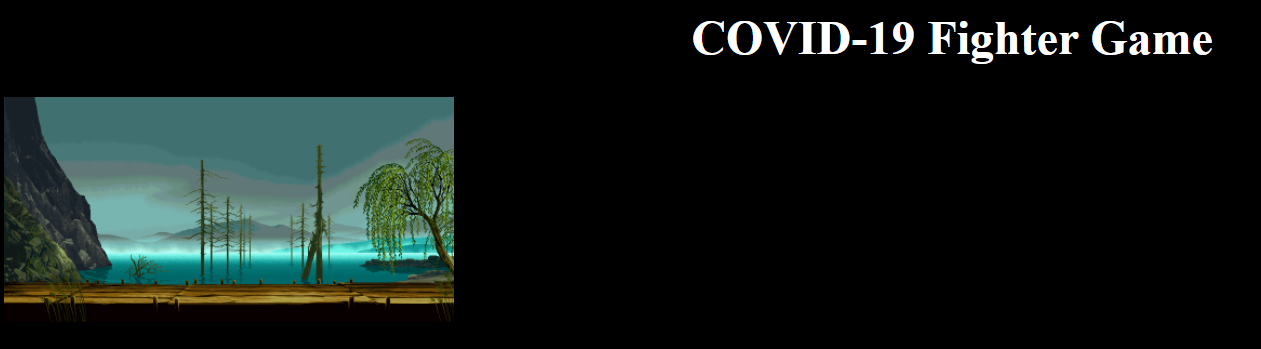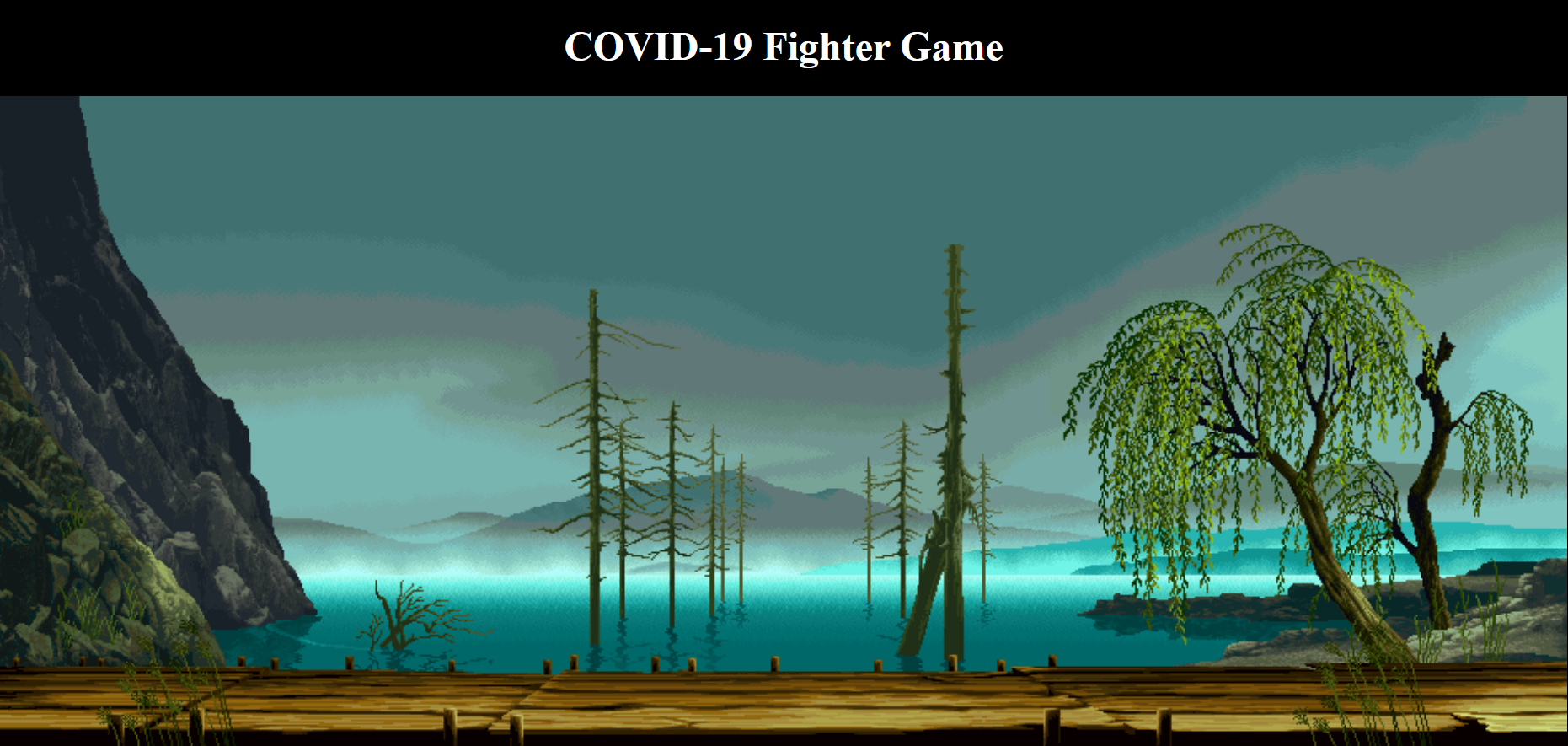# Corona Fighter Game using JavaScript

• Difficulty Level : Hard
• Last Updated : 14 Dec, 2020

In this article, we will create a covid fighter game using HTML, CSS, and JavaScript. In this game, we will create three objects the first object will represent the user which have to cross several hurdles to reach the final object.

Approach: We will create the HTML layout first, style it using CSS, and then write the logic in JavaScript. In our game, the first object represents the earth, the second represents the coronavirus, and the third represents the vaccine.

HTML code: We will use HTML to design the web page structure or layout. Create HTML canvas with id “mycanvas”. Include the JavaScript file “covid.js” in the HTML code.

## HTML

 ` ` `<``html``> ` ` `  `<``body``> ` `      ``<``h1``>COVID-19 Fighter Game ` `      ``<``canvas` `id``=``"mycanvas"``> ` `      ``<``script` `src``=``"covid.js"``> ` ` ` ` `  ` `

CSS code: CSS is used to give general styling and make it more visually appealing. Give general styling to the whole page like color, alignment, and background color. We use flex to center canvas and set the background image to the background of our game. Include the following in the above HTML code in the style section of head part of the code.

```body {
text-align: center;
color: #ffff;
background: #000;
}
#mycanvas{
display: flex;
align-items: center;
justify-content: center;
background-image: url(Assets/bg.gif)
background-size: cover;
}
```

Output: The result of the HTML layout and CSS styling looks like the following.The resultant output does not look like the desired output but we will adjust canvas height and width using JavaScript.

Logic: The main logic part is implemented using JavaScript.

• According to our logic when the earth object collides with the coronavirus (the second object ) we will decrease the health of the player by 50 points.
• The initial health of the player will be 100.
• When the health of the player will be <= 0 our game will get over.
• When the player will reach the third object player wins.
• Our game is basically divided into basic six functions.
```function load_assets(){
}
function init(){
}
function isOverlap(rect1, rect2){
}
function draw(){
}
function update(){
}
function gameloop(){
}
```

Step 1:

• Initially, we will load all the required assets. We will require one enemy, one player, winning sound, losing sound and a third object which is vaccine.
• We will create the function load_assets() which will load all the required assets.

## JavaScript

 `function` `load_assets(){ ` ` `  `    ``// Enemy object ` `    ``enemy_img = ``new` `Image(); ` `    ``enemy_img.src = ``"Assets/enemy.png"``; ` `     `  `    ``// Main user ` `    ``player_img = ``new` `Image(); ` `    ``player_img.src = ``"Assets/fighter.png"``; ` `     `  `    ``// Vaccine ` `    ``gem_img = ``new` `Image; ` `    ``gem_img.src = ``"Assets/vac.gif"``; ` `     `  `    ``// Winning sound ` `    ``win = ``new` `Audio(); ` `    ``win.src = ``"Audio/won.wav"``; ` `     `  `    ``// Losing sound ` `    ``lose = ``new` `Audio(); ` `    ``lose.src = ``"Audio/dead.mp3"``; ` `}`

Step 2:

• In this step, we will initialize our state of the game. We will set the height and width of the canvas.
• We will also set the number of second objects. We will create five enemies by setting their general position, speed,height and width.
• We will also create the player object with properties like position, height, width, speed health, and moving state.
• Create a gem object which will represent the final state of our game with properties like height, width and positions.
• At the end, add the event listener with mousedown and mouseup events to move the player and stop the player.
• We are ready with the initial setup. Let us look at the output below.

## JavaScript

 `function` `init(){ ` `    ``cvs = document.getElementById(``'mycanvas'``); ` ` `  `    ``// Setting the height and width of canvas ` `    ``W = cvs.width = 1252; ` `    ``H = cvs.height = 516; ` ` `  `    ``// Getting the context the of canvas ` `    ``pen = cvs.getContext(``'2d'``); ` ` `  `    ``// Initially setting the variable to false ` `    ``game_over = ``false``; ` ` `  `    ``// Creating the enemies object with ` `    ``// coordinates x y width(w) height(h) ` `    ``// and speed   ` ` `  `    ``e1 = { ` `        ``x:200, ` `        ``y:50, ` `        ``w:80, ` `        ``h:80, ` `        ``speed:20, ` `    ``}; ` `     ``e2 = { ` `        ``x:450, ` `        ``y:150, ` `        ``w:80, ` `        ``h:80, ` `        ``speed:35, ` `    ``};     ` `    `  `     ``e3 = { ` `        ``x:700, ` `        ``y:300, ` `        ``w:80, ` `        ``h:80, ` `        ``speed:40, ` `    ``}; ` `    ``e4 = { ` `        ``x:900, ` `        ``y:100, ` `        ``w:80, ` `        ``h:80, ` `        ``speed:30, ` `    ``}; ` `     `  `    ``// Array of enemies ` `    ``enemy = [e1, e2, e3, e4]; ` `     `  `    ``// Creating the player object ` `    ``player = { ` `        ``x:20, ` `        ``y:H/2, ` `        ``w:110, ` `        ``h:110, ` `        ``speed:30, ` `        ``health:100, ` `        ``moving: ``"false"` `    ``} ` `    ``// Creating the vaccine gem ` `    ``gem = { ` `        ``x:W-150, ` `        ``y:H/2, ` `        ``w:150, ` `        ``h:150, ` `    ``} ` ` `  `    ``// The main part lets move the player ` `    ``// using event mouse down and stop  ` `    ``//using mouse up ` `    ``cvs.addEventListener(``'mousedown'``, ``function``(){ ` `        ``console.log(``"Mouse Pressed"``);  ` `        ``player.moving = ``true``; ` `    ``}); ` `    ``cvs.addEventListener(``'mouseup'``, ``function``(){ ` `        ``console.log(``"Mouse Released"``);  ` `        ``player.moving = ``false``; ` `    ``}); ` `} `

Output:Step 3:

• In this step, we will see the overlap function which we will use to check if our player is colliding with some other object may be an enemy or a gem(vaccine).

## JavaScript

 `function` `isOverlap(rect1, rect2) { ` `    ``if` `(rect1.x < rect2.x + rect2.w && ` `        ``rect1.x + rect1.w > rect2.x && ` `        ``rect1.y < rect2.y + rect2.h && ` `        ``rect1.y + rect1.h > rect2.y) { ` `            ``return` `true``; ` `    ``} ` `    ``return` `false``; ` `} `

Step 4:

• We will see the draw() function which will help in drawing graphical images of the enemy player and gem in their respective position.

## JavaScript

 `function` `draw() { ` ` `  `    ``// Drawing the images ` `    ``for``(let i = 0; i < enemy.length; i++) { ` `        ``pen.drawImage(enemy_img, enemy[i].x,  ` `          ``enemy[i].y, enemy[i].w, enemy[i].h); ` `    ``} ` ` `  `    ``// Draw the player ` `    ``pen.drawImage(player_img, player.x,  ` `        ``player.y, player.w, player.h); ` ` `  `    ``// Draw the vaccine ` `    ``pen.drawImage(gem_img, gem.x,  ` `        ``gem.y, gem.w, gem.h); ` ` `  `    ``// Displaying score ` `    ``pen.fillStyle = ``"white"``; ` `    ``pen.font = ``"30px Roboto"``; ` `    ``pen.fillText(``"Score "` `+  ` `        ``player.health, 30, 30); ` `} `

Step 5:

• The following implements the update() function.
• It returns when the game is over.
• If the player is still moving, it will increase the player’s speed and health.
• If our player is colliding with any of the enemies, it will play the losing sound and decrease the health by 50 points.
• If health goes negative or 0, the game is over and it returns.
• If the game is still not over we will see if our player is colliding with the vaccine(gem). In this case the game is over which plays the winning sound, and alert the score.
• If the game is still not over, it will adjust the speed and positions of the enemy.

## JavaScript

 `function` `update() { ` ` `  `    ``// If player is moving ` `    ``if``(game_over){ ` `        ``return``; ` `    ``} ` `    ``if``(player.moving == ``true``){ ` `        ``player.x += player.speed; ` `        ``player.health += 20; ` `    ``} ` ` `  `    ``// Checking collision of player ` `    ``// with all enemies ` `    ``for``(let i = 0; i < enemy.length; i++){ ` `        ``if``(isOverlap(enemy[i], player)){ ` `            ``lose.play(); ` `            ``player.health -= 50; ` `            ``if``(player.health < 0){ ` `                ``draw(); ` `                ``lose.play(); ` `                ``alert(``"You Lose "``); ` `                ``game_over = ``true``; ` `                ``return``; ` `            ``} ` `        ``} ` `    ``} ` ` `  `    ``// checking the player and vaccine ` `    ``// collision for the win. ` `    ``if``(isOverlap(player, gem)){ ` `        ``win.play(); ` `        ``alert(``"You Won "` `+ player.health); ` `        ``game_over = ``true``; ` `        ``return``; ` `    ``} ` ` `  `    ``// Adjusting the speed and positions ` `    ``// of enemy ` `    ``for``(let i = 0; i H-enemy[i].h || enemy[i].y <= 0){ ` `            ``enemy[i].speed *= -1; ` `        ``} ` `    ``} ` `}`

Step 6:

• Let us complete the gameloop() function which we will use to run the game.
• If the state of the game is over, it will clear the whole interval which we are calling in the end.
• We will draw the object and update the things according to the user’s action.

## JavaScript

 `function` `gameloop(){ ` `    ``if``(game_over){ ` `        ``clearInterval(f); ` `    ``} ` `    ``draw(); ` `    ``update(); ` `} `

Step 7:

• Note: We call all the functions together in the JavaScript file “covid.js”.
• First, we will call the load_assets() and init() function.
• We will call the gameloop() function in an interval of every 100ms.

## JavaScript

 `load_assets(); ` `init(); ` `var` `f = setInterval(gameloop, 100); `

Output:

Source Code:

`https://github.com/Nandini-72/Covid_Fighter_Game`

My Personal Notes arrow_drop_up
Recommended Articles
Page :